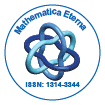Mathematica EternaOpen Access

ISSN: 1314-3344+32-466902147

Abstract

Methods for Understanding Turing Machine Computations

John Nixon

This paper is an ab initio investigation into ways of describing Turing Machine (TM) behaviour. It is shown how results for a TM restricted to a finite length of tape can be used to speed up any TM computation. The non-redundant set of such rules is referred to as the irreducible regular (computation) rules (IRR) and an algorithm is described to generate them for any TM for an arbitrary length of tape. This algorithm has been implemented in C++ as is freely available. The examples studied show that the IRR can be either finite or infinite in number. For several TM’s when they are infinite, recursive formulae for them were found and it is expected that this is always possible. These formulae were found by examining the IRR in each example separately and correctly guessing it and proving it by induction. A table showing which IRR follow others dependent on the next symbol read, was found for the examples studied and gives much information on the TM behaviour. It is anticipated that it will be possible in this way to analyse a universal TM to discover how the interpretation cycle works.

Top